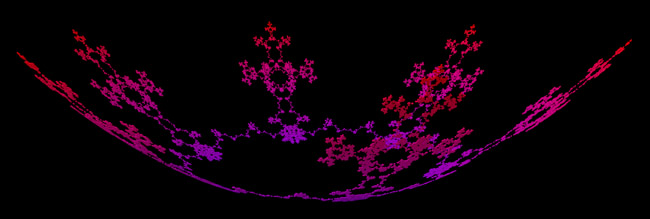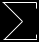# Fractal Crown

Graphics by Paul Bourke
June 2003

Equations by Roger Bagula14 w(t) =[ ei ak (-1)k t ] / [ ab k ]              -pi <= t <= pi k = 1

A 3D model is created by the following mappings

p.x = Re(w(t))
p.y = Im(w(t))
p.z = || w(t) ||2

where a = 5, b = log(2) / log(3)